Courses

# Formula Sheet and Example - Matrices and Determinants B Com Notes | EduRev

## B Com : Formula Sheet and Example - Matrices and Determinants B Com Notes | EduRev

The document Formula Sheet and Example - Matrices and Determinants B Com Notes | EduRev is a part of the B Com Course Business Mathematics and Statistics.
All you need of B Com at this link: B Com

• A + B = B + A
• (A + B) + C = A + (B + C)
• k (A + B) = kA + kB

Multiplication of matrices

• AB ≠ BA
• (AB) C = A (BC)
• Distributive law
A (B + C) = AB + AC
(A + B) C = AC + BC
• Multiplicative identity
For a square matrix A
AI = IA = A

Proerties of transpose of matrix

• (AT) T = A
• (kA)T = kAT
• (A + B)T = AT + BT
• (AB)T = BT AT

Symmetric and Skew Symmetric matrices

• Symmetric Matrix - If AT = A
• Skew - symmetric Matrix - If AT = A
Note: In a skew matrix, diagonal elements are always 0.
• For any square matrix A,
(A + AT) is a symmetric matrix
(A − AT) is a skew-symmetric matrix

Inverse of a matrix
For a square matrix A, if
AB = BA = I
Then, B is the inverse of A
i.e. B = A−1
We will find inverse of a matrix by

• Elementary transformation

Properties of Inverse

1. For a matrix A,
A1 is unique, i.e., there is only one inverse of a matrix
2. (A −1) −1 = A
3. (𝑘 𝐴)−1 = 1/𝑘 𝐴 −1
Note: This is different from
(kA)T = k AT
4. (A -1) T = (AT)-1
5. (A+B)-1 = A-1 + B-1
6. (𝐴𝐵)−1 = 𝐵−1 𝐴−1

Important things to note in Determinants

1. Determinant of Identity matrix = 1
det (I) = 1
2. |AT | = |A|
3. |AB| = |A| |B|
4. |A −1 | = 1/|𝐴|
5. |kA| = kn |A| where n is order of matrix
6. Similarly,
|−A| = |−1 × A|
= (−1)n × |A|
|"adj A| = |A | 𝑛−1
where n is the order of determinant

Number multiplied to matrix and determinant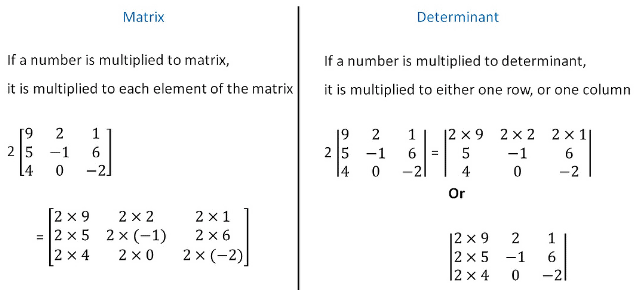Problems and Solution.
Problems 1.
Let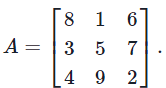Notice that A contains every integer from 1 to 9 and that the sums of each row, column, and diagonal of A are equal. Such a grid is sometimes called a magic square. Compute the determinant of A.
Solution. We compute using the first row cofactor expansion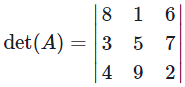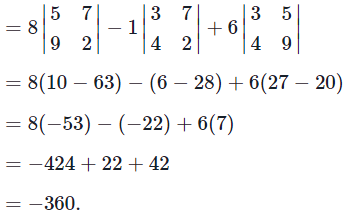Problems 2. Let A and B be n×n matrices, where n is an integer greater than 1. Is it true that det(A+B) = det(A) + det(B)?
If so, then give a proof. If not, then give a counterexample.
Solution.
We claim that the statement is false.
As a counterexample, consider the matrices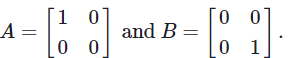Then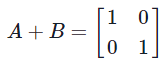and we have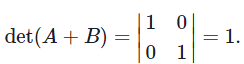On the other hand, the determinants of A and B are
det(A) = 0 and det(B) = 0,
and hence det(A) + det(B) = 0 ≠ 1 = det(A+B).
Therefore, the statement is false and in general we have
det(A+B) ≠ det(A) + det(B).

Problems 3. Let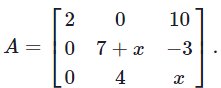Find all values of x such that A is invertible.
Solution.A matrix is invertible if and only if its determinant is non-zero.
So we first calculate the determinant of the matrix A.
By the first column cofactor expansion, we have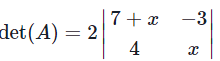= 2((7 + x)x − (−3)4) = 2(x+ 7x + 12)
= 2(x + 3)(x + 4).
Thus the determinant of A is zero if and only if x = −3 or x = −4.
Therefore the matrix A is invertible for all x except x = −3 and x = −4.

Problems 4. Let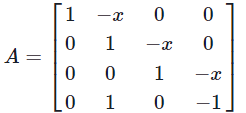be a 4×4 matrix. Find all values of x so that the matrix A is singular.
Solution. We use the fact that a matrix is singular if and only if the determinant of the matrix is zero. We compute the determinant of A as follows.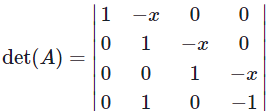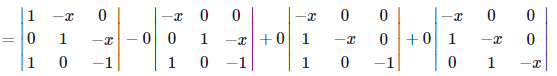by the first column cofactor expansion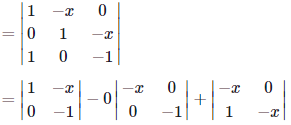by the first column cofactor expansion
= −1 + x2.
Therefore we have det(A) = x2−1. Thus det(A)=0 if and only if x = ±1.
We conclude that the matrix A is singular if and only if x = ±1.

Problems 5. Find all the values of x so that the following matrix A is a singular matrix.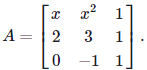Solution. Note that a matrix is singular if and only if its determinant is zero.
So we compute the determinant of the matrix A as follows.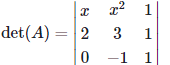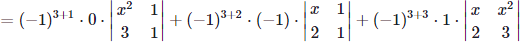by the third row cofactor expansion
= 0+(x−2)+(3x−2x2)
= −2x2+4x−2.
Thus the determinant of A is zero if
det(A) = −2x2+4x−2=0,
equivalently,
x2−2x+1=(x−1)2=0.
Thus, the determinant of the matrix A is zero if and only if x = 1.
Hence the matrix A is singular if and only if x = 1.

Problems 6. Find the value(s) of h for which the following set of vectors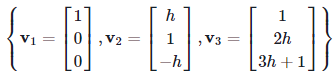is linearly independent.
Solution. Let us consider the linear combination
x1v1 + x2v2 + x3v3 = 0.     (*)
If this homogeneous system has only zero solution x1 = x2 = x3 =0, then the vectors v1,v2,v3 are linearly independent.
We reduce the augmented matrix for the system as follows.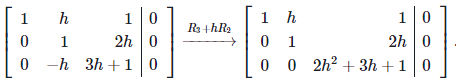From this, we see that the homogeneous system (*) has only the zero solution if and only if
2h+ 3h + 1 ≠ 0.
Since we have
2h2+3h+1=(2h+1)(h+1),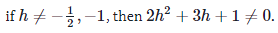In summary, the vectors v1,v2,v3 are linearly independent for any h except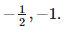Problems 7. Let A be a 3×3 matrix.
Let x,y,z are linearly independent 3-dimensional vectors. Suppose that we have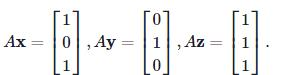Then find the value of the determinant of the matrix A.
Solution. Let B be the 3×3 matrix whose columns are the vectors x,y,z, that is,
B = [xyz].
Then we have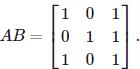Then we have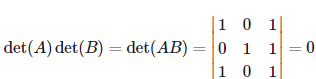(If two rows are equal, then the determinant is zero. Or you may compute the determinant by the second column cofactor expansion.)
Note that the column vectors of B are linearly independent, and hence B is nonsingular matrix. Thus the det(B)≠0.

Therefore the determinant of A must be zero.

Problems 8.  Given any constants a,b,c where a≠0, find all values of x such that the matrix A is invertible if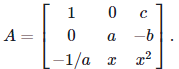Solution.
We know that A is invertible precisely when det(A)≠0. We therefore compute, by expanding along the first row,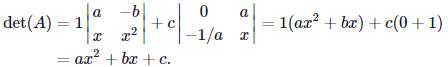Thus det(A)≠0 when ax2+bx+c≠0. We know by the quadratic formula that ax2+bx+c=0 precisely when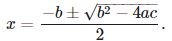Therefore, A is invertible so long as x satisfies both of the following inequalities: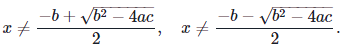Offer running on EduRev: Apply code STAYHOME200 to get INR 200 off on our premium plan EduRev Infinity!

122 videos|142 docs

,

,

,

,

,

,

,

,

,

,

,

,

,

,

,

,

,

,

,

,

,

;